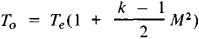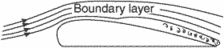# boundary layer

(redirected from Boundary layer flow)
Also found in: Dictionary, Thesaurus, Medical.
Related to Boundary layer flow: Turbulent Boundary Layer

## boundary layer

[′bau̇n·drē ‚lā·ər]
(meteorology)
The lower portion of the atmosphere, extending to a height of approximately 1.2 miles (2 kilometers).

## Boundary Layer

in a viscous fluid the flow region that forms at the surface of a body past which the fluid moves or at the interface of two streams of fluids with different velocities, temperatures, or chemical compositions; its transverse thickness is much smaller than its longitudinal dimensions. The boundary layer is characterized by an abrupt change in the transverse direction of velocity (a hydrodynamic boundary layer), temperature (a thermal boundary layer), or concentrations of individual chemical components (a diffusion boundary layer). The viscosity, thermal conductivity, and diffusivity of the fluid are the principal influences on the formation of the flow in a boundary layer. Within a dynamic boundary layer a smooth change in velocity occurs from the velocity of the external stream to zero at the wall as a result of the adhesion of the viscous fluid to the solid surface. Similarly, the temperature and concentration vary smoothly within a boundary layer.

The state of flow in a dynamic boundary layer depends on the Reynolds number Re and may be laminar or turbulent. In laminar flow, individual particles of the fluid follow trajectories whose shape is close to that of the body the fluid is flowing past or of the interface between the two liquid or gaseous media. In turbulent flow, the random, pulsating motion of individual particles of the fluid is superimposed on the average motion of the fluid particles in the direction of the main stream. The rate of transfer of momentum and the intensity of the processes of heat and mass transfer consequently are sharply increased, and an increase in skin friction and heat and mass transfer results. The value of the critical Reynolds number, at which the transition from laminar flow to turbulent flow occurs in the boundary layer, depends on several factors, including the degree of roughness of the surface past which the fluid is moving, the level of turbulence of the external stream, and the Mach number M. Moreover, the transition from laminar to turbulent flow with increasing Re in the boundary layer does not occur abruptly; there is a transition region where laminar and turbulent flows alternate.

The thickness δ of a hydrodynamic boundary layer is defined as the distance from the surface of the body (or from the interface of fluids) at which the velocity in the boundary layer can be considered essentially equal to the velocity in the external stream. The value of δ depends chiefly on the Reynolds number. For laminar flow, δ ~ lRe-0.5, and for turbulent flow, δ ~ IRe~-0.2, where l is a reference length of the body.

The development of a thermal boundary layer is determined not only by the Reynolds number but also by the Prandtl number, which characterizes the relationship between the thicknesses of the dynamic and the thermal boundary layers. The Schmidt number correspondingly has an additional effect on the development of a diffusion boundary layer.

When the external stream of gas has a high velocity, a conversion of the kinetic energy of molecules in the boundary layer into thermal energy occurs. As a result, the local temperature of the gas rises. In the case of a thermally insulated surface the temperature of the gas in a boundary layer may approach the stagnation temperaturewhere Te is the gas temperature outside the boundary layer and k = Cp/cv is the ratio of the specific heats at constant pressure and volume.

The character of the flow in a boundary layer has a decisive effect on the separation of the flow from the surface of the body past which the fluid is moving. The reason for this is that when a sufficiently great positive longitudinal pressure gradient is present, the kinetic energy of the fluid particles that are slowed down in the boundary layer becomes insufficient to overcome the pressure forces, the flow in the boundary layer loses stability, and separation of flow occurs.

When the Reynolds numbers are very large, the thickness of the boundary layer is very small in comparison with the characteristic dimensions of the body. Throughout nearly the entire flow region, with the exception of the thin boundary layer, the effect of viscous forces is therefore insignificant in comparison with inertial forces, and the fluid in this region can be regarded as perfect. At the same time, because of the small thickness of the boundary layer, the pressure in it in a transverse direction can be considered essentially constant. As a result, a highly effective method of studying the flow of a fluid stream past a body is the division of the entire flow field into two parts—the flow region of the perfect fluid and the thin boundary layer at the surface of the body. The flow in the first region is studied by means of the equations of motion of a perfect fluid; this approach permits determination of the pressure distribution along the surface of the body. The pressure in the boundary layer is also thereby determined. The flow within the boundary layer is then calculated, taking into account viscosity, thermal conductivity, and diffusion. As a result, the surface friction and the coefficients of heat and mass transfer can be determined. This approach, however, is inapplicable in its explicit form in the case of flow separation from the surface of the body. It also is inapplicable for small Re, when the effect of viscosity extends to quite large distances from the surface of the body.

### REFERENCES

Loitsianskii, L. G. Mekhanika zhidkosti i gaza, 4th ed. Moscow, 1973.
Schlichting, H. Teoriia pogranichnogo sloia. Moscow, 1974. (Translated from German.)
Osnovy teploperedachi v aviatsionnoi i raketnoi tekhnike. Moscow, 1960.
Kutateladze, S. S., and A. I. Leont’ev. Teplomassoobmen i trente v turbulentnom pogranichnom sloe. Moscow, 1972.

N. A. ANFIMOV

## boundary layerThe layer of fluid, close to the surface of a body placed in a moving stream, in which the impact pressure is reduced as a result of the viscosity of the fluid. A velocity gradient exists through the boundary layer, ranging from the velocity of the body to the velocity of the free airstream. The nature of the boundary layer determines the maximum lift coefficient, the stalling characteristics of a wing, the value of form drag, and to an extent the high-speed characteristics. In aerodynamics, the boundary-layer thickness is measured from the surface to an arbitrarily chosen point (e.g., where the velocity is 99% of the stream velocity). Thus, in aerodynamics, the boundary layer, by selection of the reference point, can include only the laminar boundary layer or the laminar boundary layer plus all, or a portion of, the turbulent boundary layer.
References in periodicals archive ?
Entropy Generation in MHD Free Convective Boundary Layer Flow Past an Inclined Flat Plate Embedded in a Porous Medium with Hall Currents, International Journal of Computers Applications 84(9): 36-46.
Pop, "Analytic series solution for unsteady mixed convection boundary layer flow near the stagnation point on a vertical surface in a porous medium," Transport in Porous Media, vol.
16] Sajjad Hussain, MHD Forced Convective Boundary Layer Flow of Micropolar Fluids past a Shrinking Porous Sheet Prescribed with Variable Heat Flux and Heat Source, J.
Analysis of boundary layer flow over a porous nonlinearly stretching sheet with partial slip at the boundary, Alex.
1996) Magnetohydrodynamic boundary layer flow and heat transfer on a continuous moving wavy surface, Arch.
Magnetohydrodynamic boundary layer flow for a non-Newtonian fluid past a wedge, Astrophysics and Space Science 141 (1988) 9-19.
This suggests that in comparison with the stationary flow, different processes govern the development of the boundary layer flow under breaking waves.
The equations governing the boundary layer flow of viscous incompressible fluid past a stretching plate are
From (13) choosing C = 2, the length scale [sigma](t) = 2[square root of [upsilon] t] which exactly corresponds to the usual scaling factor for various unsteady boundary layer flows (Schlichting ).
Micropolar fluids have been used by many authors in the boundary layer flow and heat transfer for different geometries under several physical and thermal conditions, for example [3-7].

Site: Follow: Share:
Open / Close